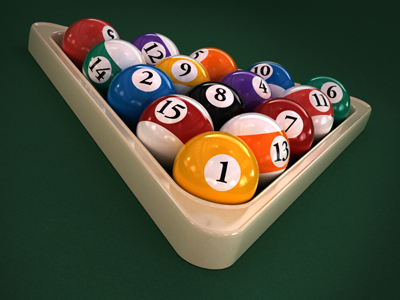This triangle used in the game of pool is an equilateral triangle.

# Shapes - Angles 1

This Math quiz is called 'Shapes - Angles 1' and it has been written by teachers to help you if you are studying the subject at middle school. Playing educational quizzes is a fabulous way to learn if you are in the 6th, 7th or 8th grade - aged 11 to 14.

It costs only \$12.50 per month to play this quiz and over 3,500 others that help you with your school work. You can subscribe on the page at Join Us

Angles are measured in degrees. Your Math teachers will no doubt be teaching you all about angles. An angle is an amount of turning or rotation.

Turn your attention to our Shapes quiz on the subject.

1.
An angle that is between 0o and 90o is a(n) .......
acute angle
obtuse angle
reflex angle
right angle
2.
An angle that is between 90o and 180o is a(n) .......
acute angle
obtuse angle
reflex angle
right angle
3.
Two interior angles in a triangle are 35o and 55o. What is the 3rd angle?
75o
85o
90o
95o
Take 35 and 55 from 180 to find the answer
4.
Angles on a straight line add up to what?
90o
180o
270o
360o
5.
An angle that is between 180o and 360o is a(n) .......
acute angle
obtuse angle
reflex angle
right angle
6.
Each internal angle of a regular pentagon is 108o. What is the total number of internal degrees?
180o
360o
540o
720o
7.
The three interior angles in a triangle add up to what?
90o
180o
270o
360o
An equilateral triangle has 3 interior angles each measuring 60 degrees each (3 x 60 = 180)
8.
An angle that is 90o is a(n) .......
acute angle
obtuse angle
reflex angle
right angle
9.
The internal angles of a regular hexagon add up to 720o. How many degrees is each one?
60o
90o
120o
150o
There are six internal angles in a hexagon. A regular hexagon has six equal angles
10.
A circle is divided into a number of parts or degrees. How many are there?
90o
180o
270o
360o
The four interior angles of a quadrilateral also add up to 360o
Author:  Frank Evans